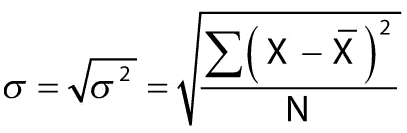# Standard deviation in R: How to Use sd() in R

We have already seen how to calculate percentile and variance in R programming. We have also seen how to calculate Mean and Mode in R. Let’s see how to compute the standard deviation, but before that, let’s understand what SD is.

## What is Standard Deviation

The standard deviation of a population is the square root of the population variance. It is the measure of the distribution of the values. The higher the standard deviation, the wider the spread of values. The lower the standard deviation, the closer the spread of values.

The standard deviation is a generally used model of the degree of variation within a set of data values. A low standard deviation relative to the mean value of a sample means the observations are tightly clustered; larger values suggest observations are more spread out.

## standard deviation in r

To calculate the standard deviation in r, use the sd() function. The standard deviation of an observation variable in R is calculated by the square root of its variance.

The sd in R is a built-in function that accepts the input object and computes the standard deviation of the values provided in the object. The sd() function accepts a numerical vector and logical arguments and returns the standard deviation.

If na.rm is TRUE, then missing values are removed before computation proceeds. If the input value is a matrix or a data frame, a vector of the standard deviation of the columns is returned.

The symbol for the population standard deviation is Σ (sigma). Its formula is the following.### Syntax

The syntax of the sd() function in R is the following.

``sd(x, na.rm = FALSE)``

### Parameters

x: It is a numeric vector or an R object but not a factor coercible to numeric by as.double(x).

na.rm: It is logical. Should missing values be removed?

### Example

We will find the standard deviation of the Petal.length of the iris dataset.

``````data(iris)
iris\$Petal.Length
ln <- iris\$Petal.Length
cat("The standard deviation of iris petal length is: ", "\n")
sd(ln)``````

#### Output

`````` 1.4 1.4 1.3 1.5 1.4 1.7 1.4 1.5 1.4 1.5 1.5 1.6 1.4 1.1 1.2 1.5 1.3 1.4
 1.7 1.5 1.7 1.5 1.0 1.7 1.9 1.6 1.6 1.5 1.4 1.6 1.6 1.5 1.5 1.4 1.5 1.2
 1.3 1.4 1.3 1.5 1.3 1.3 1.3 1.6 1.9 1.4 1.6 1.4 1.5 1.4 4.7 4.5 4.9 4.0
 4.6 4.5 4.7 3.3 4.6 3.9 3.5 4.2 4.0 4.7 3.6 4.4 4.5 4.1 4.5 3.9 4.8 4.0
 4.9 4.7 4.3 4.4 4.8 5.0 4.5 3.5 3.8 3.7 3.9 5.1 4.5 4.5 4.7 4.4 4.1 4.0
 4.4 4.6 4.0 3.3 4.2 4.2 4.2 4.3 3.0 4.1 6.0 5.1 5.9 5.6 5.8 6.6 4.5 6.3
 5.8 6.1 5.1 5.3 5.5 5.0 5.1 5.3 5.5 6.7 6.9 5.0 5.7 4.9 6.7 4.9 5.7 6.0
 4.8 4.9 5.6 5.8 6.1 6.4 5.6 5.1 5.6 6.1 5.6 5.5 4.8 5.4 5.6 5.1 5.1 5.9
 5.7 5.2 5.0 5.2 5.4 5.1

The standard deviation of iris petal length is:

 1.765298``````

That is it. The standard deviation for the petal.length is 1.765298.

You can calculate the standard deviation without the sd() function using the following code.

``sqrt(sum((ln - mean(ln)) ^ 2 / (length(ln) - 1)))``

The complete code is following.

``````data(iris)
iris\$Petal.Length
ln <- iris\$Petal.Length
cat("The standard deviation of iris petal length is: ", "\n")
sqrt(sum((ln - mean(ln)) ^ 2 / (length(ln) - 1)))``````

#### Output

`````` 1.4 1.4 1.3 1.5 1.4 1.7 1.4 1.5 1.4 1.5 1.5 1.6 1.4 1.1 1.2 1.5 1.3 1.4
 1.7 1.5 1.7 1.5 1.0 1.7 1.9 1.6 1.6 1.5 1.4 1.6 1.6 1.5 1.5 1.4 1.5 1.2
 1.3 1.4 1.3 1.5 1.3 1.3 1.3 1.6 1.9 1.4 1.6 1.4 1.5 1.4 4.7 4.5 4.9 4.0
 4.6 4.5 4.7 3.3 4.6 3.9 3.5 4.2 4.0 4.7 3.6 4.4 4.5 4.1 4.5 3.9 4.8 4.0
 4.9 4.7 4.3 4.4 4.8 5.0 4.5 3.5 3.8 3.7 3.9 5.1 4.5 4.5 4.7 4.4 4.1 4.0
 4.4 4.6 4.0 3.3 4.2 4.2 4.2 4.3 3.0 4.1 6.0 5.1 5.9 5.6 5.8 6.6 4.5 6.3
 5.8 6.1 5.1 5.3 5.5 5.0 5.1 5.3 5.5 6.7 6.9 5.0 5.7 4.9 6.7 4.9 5.7 6.0
 4.8 4.9 5.6 5.8 6.1 6.4 5.6 5.1 5.6 6.1 5.6 5.5 4.8 5.4 5.6 5.1 5.1 5.9
 5.7 5.2 5.0 5.2 5.4 5.1

The standard deviation of iris petal length is:

 1.765298``````

## Calculate the Standard deviation of Vector in R

To calculate the standard deviation of the vector, use the sd() function. To define a vector, use the c() function and pass the elements as arguments. You can also use the :(colon) operator to create a vector.

``````vec <- 1:5
cat("The standard deviation of vector is", "\n")
sd(vec)``````

#### Output

``````The standard deviation of vector is
 1.581139``````

And we get the standard deviation of the numeric vector, which in our example is 1.581139.

## Calculate standard deviation of Array in R

To calculate the standard deviation of an array in R, use the sd() function. To create an array in Ruse the array() function. The array() function takes a vector as an argument and uses the dim parameter to create an array.

``````rv <- c(19, 21)
rv2 <- c(46, 4)
arr <- array(c(rv, rv2), dim = c(2, 2, 2))
cat("The standard deviation of array is", "\n")
sd(arr)``````

#### Output

``````The standard deviation of array is
 16.11565``````

## Calculate the Standard deviation of data frame in R

To calculate the standard deviation of a data frame in R, use the sd() function. To create a data frame in R, use data.frame() function. We will find the standard deviation of a numerical column of the data frame.

``````df <- data.frame(service_id = c(1:5),
service_name = c("Netflix", "Disney+", "HBOMAX", "Hulu", "Peacock"),
service_price = c(18, 10, 15, 7, 12),
stringsAsFactors = FALSE)
cat("The standard deviation of service_price is", "\n")
sd(df\$service_price)``````

#### Output

`` 4.27785``

And we get the SD of the data frame column.

That’s it for this tutorial.